GeeksforGeeks App
Open AppBrowser
Continue

## Related Articles

Weak AVL trees, also known as rank-balanced trees, are a type of self-balancing binary search tree that aims to balance the tree while minimizing the number of rotations needed to maintain balance. These trees are similar to AVL trees in that they both use rotations to maintain balance, but they differ in how they define balance.

### Explanation:

In an AVL tree, the height difference between the left and right subtrees of any node must not exceed 1. This means that the tree is very strictly balanced, with the left and right subtrees of each node having roughly the same height. While this ensures good balance and fast search times, it also means that AVL trees require a lot of rotations to maintain balance, especially when the tree is constantly being modified by inserting or deleting elements.

• In a weak AVL tree, the left and right subtrees of a node may have very different heights, as long as the rank difference between them is not too large. This allows weak AVL trees to maintain balance with fewer rotations than regular AVL trees, which can make them more efficient in certain situations.
• One trade-off of using weak AVL trees is that they may not be as balanced as regular AVL trees, which can lead to longer search times in the worst case. However, in practice, weak AVL trees can be very effective at maintaining good balance and performance, especially in environments where the data being stored is not highly dynamic.

### Properties of Weak AVL or Rank Balanced Trees:

• In a weak AVL tree, each node is either left-heavy or right-heavy. This means that the height of the left subtree and the height of the right subtree differ by at most 1. This is in contrast to a strong AVL tree, in which the height of the left and right subtrees differ by at most 2.
• Weak AVL trees are less balanced than strong AVL trees, which means that they may have longer search times. However, they are easier to insert and delete, which makes them a good choice for certain types of applications.
• Like strong AVL trees, weak AVL trees are self-balancing binary search trees, which means that they maintain a certain level of balance automatically as elements are inserted and deleted. This makes them efficient for searching and maintaining a sorted list of elements.

Some of the key properties of weak AVL trees include:

• Every node has a balance factor of -1, 0, or 1.
• The height of the left and right subtrees of a node differ by at most 1.
• The height of the tree is O(log n), where n is the number of nodes in the tree.
• Insertion and deletion operations take O(log n) time in the worst case.

### How the tree is balanced?

In a weak AVL tree, a node is considered “weak” if the height difference between its left and right subtrees is greater than 1. If a node becomes weak, it is balanced by rotating its subtrees in a similar manner to a regular AVL tree.

There are two types of rotations that can be performed on a weak AVL tree: left rotations and right rotations

• A left rotation is performed on a node with a right-heavy subtree (i.e. the height of its right subtree is greater than the height of its left subtree).
• A right rotation is performed on a node with a left-heavy subtree (i.e. the height of its left subtree is greater than the height of its right subtree).

For example, consider the following weak AVL tree: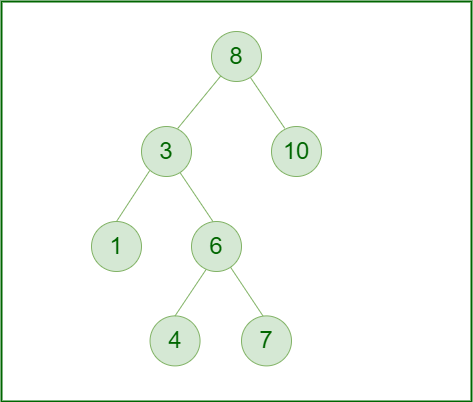Example of Weak AVL Tree

The node with value 8 is weak because the height difference between its left and right subtrees is greater than 1. To balance this tree, we can perform a right rotation on the node with value 8: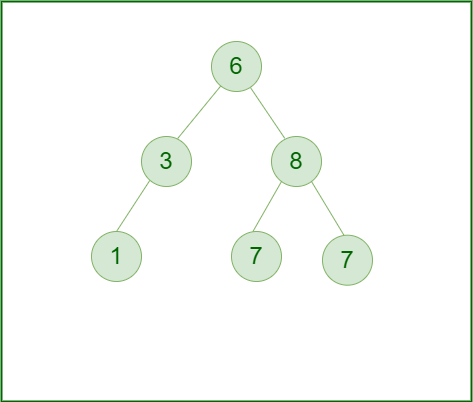Right rotation on the node with value 8

This rotates the subtree rooted at 8 to the right, and the subtree rooted at 6 to the left. This results in a balanced tree, with the height difference between the left and right subtrees of each node being less than or equal to 1.

Weak AVL trees are similar to regular AVL trees in that they are self-balancing, but they are less strict in their balancing criteria. This can make them faster to insert into and delete from, but at the cost of potentially lower performance for certain operations.

### How to implement each of the update, insertion, deletion, and searching in the Weak AVL or Rank Balanced Trees:

Here is an approach for step-by-step implementing each of the update, insertion, deletion, and search operations in a weak AVL tree or ranked balanced tree, along with their complexities:

### Update:

• Traverse the tree from the root to the node that you want to update.
• Update the value of the node.
• Traverse back up the tree, starting from the updated node.
• For each node encountered, update its balance factor (i.e., the difference in the height of its left and right subtrees).
• If the balance factor of a node becomes greater than 1 or less than -1, perform a rotation on that node to restore balance.

The complexity of the update operation is: O(log n)

### Insertion:

• Traverse the tree from the root to find the correct position for the new element.
• Insert the new element as a leaf node in the tree.
• Traverse back up the tree, starting from the inserted node.
• For each node encountered, update its balance factor (i.e., the difference in the height of its left and right subtrees).
• If the balance factor of a node becomes greater than 1 or less than -1, perform a rotation on that node to restore balance.

The complexity of the  insertion operation is: O(log n)

### Deletion:

• Traverse the tree from the root to find the node that you want to delete.
• If the node has no children, simply remove it from the tree.
• If the node has one child, remove the node and replace it with its child.
• If the node has two children, find the successor of the node (i.e., the next-largest element in the tree) and replace the node with its successor. Then delete the successor from its original position in the tree.
• Traverse back up the tree, starting from the node that was just deleted.
• For each node encountered, update its balance factor (i.e., the difference in the height of its left and right subtrees).
• If the balance factor of a node becomes greater than 1 or less than -1, perform a rotation on that node to restore balance.

The complexity of the deletion operation is: O(log n)

### Searching:

• Traverse the tree from the root, following the left or right child of each node based on whether the search key is less than or greater than the value of the node.
• If the search key is found, return the node. If the search key is not found, return null.

The complexity of the search operation is: O(log n)

### Complexity Analysis:

The time complexity of operations on a weak AVL tree or a rank-balanced tree depends on the specific operation being performed and the structure of the tree. In general, however, these trees have time complexities similar to those of regular AVL trees for most operations.

For example, the time complexity of inserting a new node into a weak AVL tree or a rank-balanced tree is typically O(log n), where n is the number of nodes in the tree. This is because these trees are self-balancing and maintain a logarithmic height, similar to regular AVL trees.0

• The time complexity of searching for a specific node in a weak AVL tree or a rank-balanced tree is also typically O(log n). This is because these trees are structured in a way that allows for efficient search by repeatedly dividing the search space in half at each level.
• The Time complexity of deleting a node from a weak AVL tree or a rank-balanced tree is also typically O(log n), as the tree must be rebalanced after the deletion to maintain its self-balancing properties.

Overall, the time complexity of operations on a weak AVL tree or a rank-balanced tree is similar to that of a regular AVL tree, but with potentially lower performance for certain operations due to the relaxed balancing criteria.

### How does the rebalancing happen to Weak AVL or Rank Balanced Trees?

In a weak AVL tree or ranked balanced tree, rebalancing is performed using rotations. A rotation is a local operation that rearranges the nodes in the tree to restore balance. There are two types of rotations: left rotations and right rotations.

A left rotation is performed on a node that is right-heavy, meaning that the height of its right subtree is greater than the height of its left subtree.

• To perform a left rotation, the right child of the node becomes the new root of the subtree, and the original root becomes the left child of the new root. The left subtree of the new root and the right subtree of the original root is unaffected by the rotation.

Right rotation is performed on a node that is left-heavy, meaning that the height of its left subtree is greater than the height of its right subtree.

• To perform a right rotation, the left child of the node becomes the new root of the subtree, and the original root becomes the right child of the new root. The right subtree of the new root and the left subtree of the original root is unaffected by the rotation.

In a weak AVL tree or ranked balanced tree, rotations are used to maintain balance after insertions and deletions. When a new element is inserted into the tree, it may cause the tree to become unbalanced. To restore balance, the tree is traversed from the inserted node up to the root, and rotations are performed as needed to restore the balance conditions. Similarly, when an element is deleted from the tree, it may cause the tree to become unbalanced. In this case, rotations are also used to restore balance.

### Here is an example of how rebalancing happens in a weak AVL tree or ranked balanced tree:

Suppose we have the following weak AVL tree: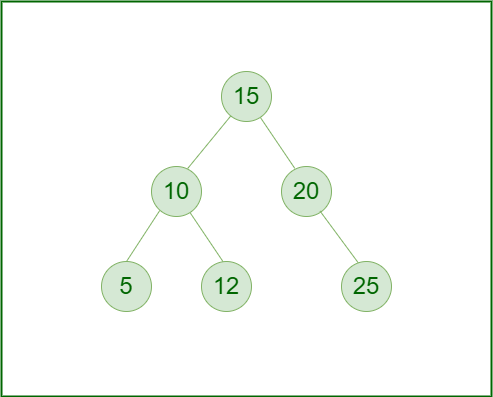Example of Weak AVL tree

Now suppose we want to insert element 13 into this tree. We first insert it as we would in a standard binary search tree, by finding the correct position for it based on its value. In this case, we would insert it as the right child of the node with value 12: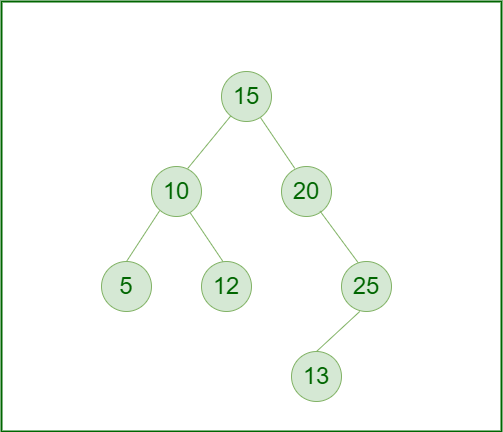Insertion of 13 in WAVL Tree

Now the tree is no longer balanced, because the right subtree of the node with value 12 has a height of 2, while the left subtree has a height of 0. To restore balance, we perform a left rotation on the node with value 12: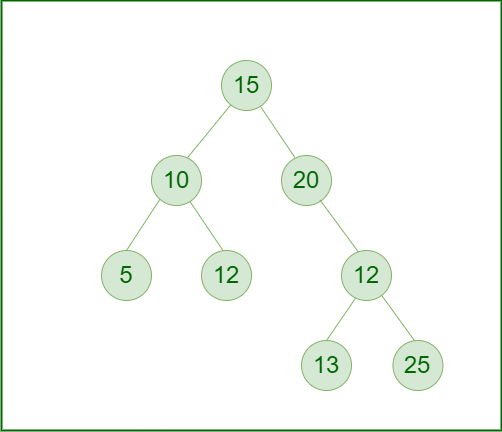Left Rotation on the WAVL Tree

Now the tree is balanced again because the height of the left and right subtrees of each node differ by at most 1.

### The difference with a height-balanced tree:

A height-balanced tree is a type of self-balancing binary search tree in which the height difference between the left and right subtrees of any node is limited to a specific value, usually 1 or 2. This helps to ensure that the tree remains balanced, with a logarithmic height, which allows for efficient insertion, deletion, and search operations.

• A weak AVL tree is a type of height-balanced tree that is similar to a regular AVL tree, but with less strict balancing criteria. In a weak AVL tree, a node is considered “weak” if the height difference between its left and right subtrees is greater than 1. If a node becomes weak, it is balanced by rotating its subtrees in a similar manner to a regular AVL tree.
• A rank-balanced tree is another type of height-balanced tree that is similar to a weak AVL tree but with a different balancing criterion. In a rank-balanced tree, a node is considered “unbalanced” if the number of nodes in its left or right subtree differs from the number of nodes in the other subtree by more than a specified rank threshold. If a node becomes unbalanced, it is balanced by rotating its subtrees in a similar manner to a regular AVL tree.

My Personal Notes arrow_drop_up